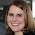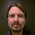## Wednesday, January 31, 2018

### Statistical Sins: Types of Statistical Relationships

On this blog, I've covered a lot of statistical topics, and have tried to make them approachable even to people with little knowledge of statistics. But I admit that, recently, I've been covering more advanced topics. But there are still many basic topics to explore, that could be helpful for non-statistical readers, as well as teachers of statistics who address these topics in courses. This topic was prompted by a couple of discussions - one late last week, and another at dance class last night.

The discussions dealt with what it means to say two variables are related to each other (or not), including whether that relationship is weak, moderate, or strong, and whether it is positive or negative. I first addressed this topic when I wrote about correlation for my 2017 April A to Z. But let's really dive into that topic.

You may remember that correlation ranges from -1 to +1. The closer the value is to 1 (positive or negative), the stronger the relationship between the two variables. So in terms of strength, it is the absolute value that matters. A correlation close to 0 indicates no relationship between the variables.

A common question I see is whether a correlation is weak, moderate, or strong. Different people use different conventions, and there isn't a lot of agreement on this topic, but I frequently see a correlation of 0.5 referred to as strong. As I explained in my post on explained variance, this means the two variables share about 25% variance, or more specifically, about 25% of the variation in one variable can be explained by the other variable.

For moderate, I often see 0.3 (or 9% shared variance) and for weak, 0.1 (or 1% shared variance). But as I said, these really aren't established conventions (please don't @ me - thanks in advance). You could argue that there are a variety of factors that influence whether a relationship is seen as weak, moderate, or strong. For instance, study methods could have an impact. Finding a correlation of 0.5 between two variables I can directly manipulate and/or measure in an experimental setting is completely different from finding a correlation of 0.5 between two variables simply measured in a natural setting, where I have little to no control over confounds and high potential for measurement error. And we are often more generous about what we consider strong or weak in new areas of research than in well-established topics. But conventions give you a nice starting point, that you can then shift as needed depending on these other factors.

Direction of the relationship is indicated by the sign. A positive correlation means a positive relationship - as scores on one variable go up, so do scores on the other variable. For example, calories consumed per day and weight would be positively correlated.

A negative correlation means a negative relationship - as scores on one variable go up, scores on the other variable go down. For instance, minutes of exercise per day and weight would probably have a negative correlation.

[Now, for that last relationship, you might point out that there are a variety of other factors that could change that relationship. For example, some exercises burn fat, lowering weight, while others build muscle, which might increase weight. I hope to explore this topic a bit more later: how ignoring subgroups in your data can lead you to draw the wrong conclusions.]

Part of the confusion someone had in our discussion last night was knowing the difference between no relationship and a negative relationship. That is, they talked about how one variable (early success) had no bearing on another variable (future performance). They quickly pointed that this doesn't mean having early success is bad - the relationship isn't negative. But I think there is a tendency for people unfamiliar with statistics to confuse "non-predictive" with "bad".

So let's demonstrate some of these different relationships. To do that, I've generated a dataset in R, using the following code to force the dataset to have specific correlations. (You can use the code to recreate on your own.) The first thing I do is create a correlation matrix, which shows the correlations between each pairing of variables, that reflect a variety of relationship strengths and directions. Then, I impose that correlation matrix onto a randomly generated dataset. Basically R generates data that produces a correlation matrix very similar to the one I defined.

R1 = c(1,0.6,-0.5,0.31,0.11)
R2 = c(0.6,1,-0.39,-0.25,0.05)
R3 = c(-0.5,-0.39,1,-0.001,-0.09)
R4 = c(0.31,-0.25,-0.001,1,0.01)
R5 = c(0.11,0.05,-0.09,0.01,1)

R = cbind(R1,R2,R3,R4,R5)
U = t(chol(R))
nvars = dim(U)
numobs = 1000
set.seed(36)
random.normal = matrix(rnorm(nvars*numobs,0,1),nrow = nvars, ncol=numobs)
X = U %*% random.normal
newX = t(X)
raw = as.data.frame(newX)
names(raw) = c("V1","V2","V3","V4","V5")
cor(raw)

The final command, cor, which requests a correlation matrix for the dataset, produces the following:

V1          V2         V3          V4          V5
V1  1.0000000  0.57311834 -0.4629099  0.31939003  0.10371136
V2  0.5731183  1.00000000 -0.3474012 -0.26425660  0.04838563
V3 -0.4629099 -0.34740123  1.0000000  0.01204920 -0.12017036
V4  0.3193900 -0.26425660  0.0120492  1.00000000  0.01202121
V5  0.1037114  0.04838563 -0.1201704  0.01202121  1.00000000

Just looking down the columns, you can see that the correlations are very close to what I specified. The one exception is the correlation between V3 and V4 - I asked for -0.001 and instead have 0.012. Probably, R couldn't figure out how to generate data with that correlation while also coming close to the other values I specified. So it had to fudge this one a bit.

So now, I can plot these different relationships in scatterplots, to let you see what weak, moderate, and strong relationships look like, and how direction changes the appearance. Let's start by looking at our weak correlations, positive and negative. (Code below, including lines to add a title and center it.)

library(ggplot2)

weakpos<-ggplot(raw,aes(x=V1,y=V5)+geom_point()
+labs(title="Weak Positive")
+theme(plot.title=element_text(hjust=0.5))

weakneg<-ggplot(raw,aes(x=V3,y=V5)+geom_point()
+labs(title="Weak Negative")
+theme(plot.title=element_text(hjust=0.5))

That code produces these two plots:

With similar code (just switching out variables in the x= and y= part), I can produce my moderate plots:

and my strong plots:

As you can see, even a strong relationship looks a bit like a cloud of dots, but you can see trends that go from almost nonexistent to more clearly positive or negative. You can make the trends a bit easier to spot by adding a trendline. For example:

strongpos+geom_smooth(method=lm)

The flatter the line, the weaker relationship. Two variables that are unrelated to each other (such as V4 and V5) will have a horizontal line through the scatter:

I'll come back to this topic Sunday (and in the future post idea I mentioned above), so stay tuned!

1.Hello,
I really enjoy the simplicity and clarity of your posts dealing with unpacking foundational stats concepts. I teach a bit of research methods and made some Shiny apps to demo some similar concepts (here: https://petemiksza.com/visualizing-statistical-concepts/). Maybe the correlation app would be of interest? Also, would appreciate any feedback for improvement if you had any.
Thanks for these posts!
Pete

1.Awesome! Thank so much for sharing, Pete! I'll check out your apps and let you know if I have any thoughts/feedback. Mind if I add you to my list of Data Science and Statistics resources? http://www.deeplytrivial.com/2017/10/statistics-sunday-free-data-science-and.html

2.2."Just looking down the columns, you can see that the correlations are very close to what I specified. The one exception is the correlation between V3 and V4 - I asked for -0.001 and instead have 0.012. Probably, R couldn't figure out how to generate data with that correlation while also coming close to the other values I specified." Not really, that correlation one is 'as off' as most of the rest. It's just sampling error: Calculate the differences between the unique, off-diagonal elements in R and cor(raw) and plot a histogram. You'll obtain a distribution around 0 (which would approximate a normal distribution if the matrices are large).

If you want to generate exact data (i.e. population data rather than sample data), you can make use the function mvrnorm (in library MASS), for instance.

An example, (run after installing MASS):

R <- matrix (scan(), 5, 5, TRUE)
1 0.6 -0.5 0.31 0.11
0.6 1 -0.39 -0.25 0.05
-0.5 -0.39 1 -0.001 -0.09
0.31 -0.25 -0.001 1 0.01
0.11 0.05 -0.09 0.01 1

set.seed(36)

nvars <- ncol( R )

nobs <- 1000

raw <- MASS::mvrnorm( nobs, rep ( 0, nvars ), R, emp = TRUE )

cor( raw )

# change emp = TRUE to emp = FALSE (the default) in order tot generate sample data

3.Thanks for the tip how to plot trend lines!